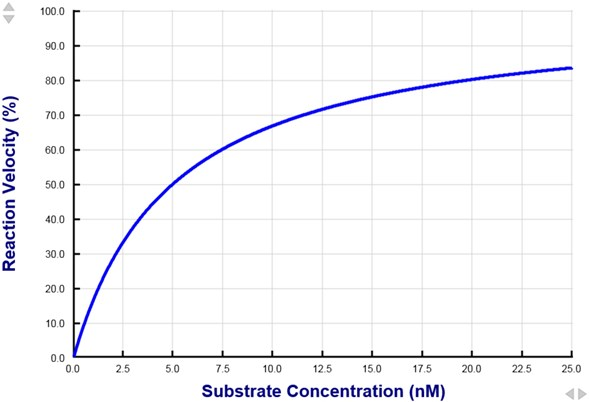PhysiologyWeb  Home  |  FAQ  |  About  |  Contact  |   - Sign InPhysiology Quiz -
Michaelis-Menten Kinetics - Part 2
Michaelis-Menten kinetics is used to describe the rate of a reaction catalyzed by an enzyme as a function of the substrate concentration. This type of analysis is also commonly used to describe the rate of transporter-mediated translocation of ions and molecules across biological membranes. Test your basic knowledge of Michaelis-Menten kinetics by taking this simple quiz.
(1)   A higher numerical value of the Michaelis constant (Km) indicates a lower enzyme/transporter affinity for its substrate.
 True False
(2)   The figure below shows the reaction velocity catalyzed by an enzyme as a function of the enzyme substrate concentration. Such a relationship is typically referred to as a dose-response relationship. Based on the data shown, what is the Km (Michaelis constant) of this enzyme for its substrate? Note that the reaction velocity is normalized to the maximum velocity (Vmax). Therefore, in the plot shown, Vmax = 100%.(A)   2.5 nM (B)   5.0 nM (C)   10.0 nM (D)   20.0 nM (E)   25.0 nM
(3)   Assume that the reaction catalyzed by an enzyme follows Michaelis-Menten kinetics. The substrate concentration (Km, Michaelis constant) needed to reach 50% of the maximum reaction velocity (Vmax) is 25 μM. What substrate concentration is required to obtain at least 50% of the maximum reaction velocity?
 (A)   25 μM (B)   50 μM (C)   100 μM (D)   250 μM (E)   475 μM
(4)   The Michaelis-Menten equation represents a special case of the Hill equation, where the Hill coefficient has been set to one.
 True False
(5)   Assume that the reaction catalyzed by an enzyme follows Michaelis-Menten kinetics. If at a substrate concentration of 100 nM, the reaction proceeds at 50% of the maximum reaction velocity (Vmax), what is the Michaelis constant (Km) for this substrate. Km is the substrate concentration needed to reach 50% of Vmax.
 (A)   25 nM (B)   50 nM (C)   100 nM (D)   150 nM (E)   200 nM